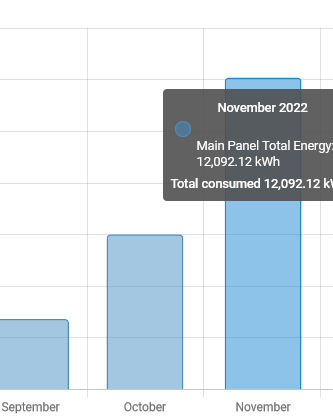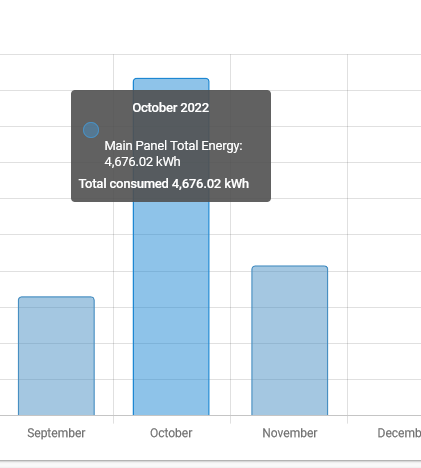## Rewriting Home Assistant Long-term statistics from InfluxDB

In an earlier post, I made an error that incorrectly aggregated the energy data which resulted in hugely inflated aggregated energy usage. All the un-aggregated data was accurate, but the sums were wrong. Luckily I had all the raw data stored in InfluxDB and could rebuild it.

In this post, I walk through how to re-write the Home Assistant Long-term statistics database to fix this mistake.A grossly high electric bill

First, I opened up the Influx UI and constructed a query to generate the corrected metrics. The HA database requires two fields: sum and state. One describes the cumulative running sum, and the other is the sum at the end of the hour. I didn’t fully understand how sum differed from state, so I just assumed they were the same.

 `````` 1 2 3 4 5 6 7 8 9 10 `````` ``````from(bucket: "energy-1h") |> range(start: -365d, stop: now()) |> filter(fn: (r) => r["_measurement"] == "kWh") |> filter(fn: (r) => r["_field"] == "value") |> filter(fn: (r) => r["domain"] == "sensor") |> filter(fn: (r) => r["entity_id"] != "main_panel_total_energy" and r["entity_id"] != "sub_panel_32_total_power" and r["entity_id"] != "daily_electricity_usage") |> group(columns: ["_measurement", "_field"]) |> aggregateWindow(every: 1h, fn: sum, createEmpty: false) |> cumulativeSum() |> yield(name: "mean") ``````

That gave me a file that looked like the following:

 ``````1 2 3 4 5 6 `````` ``````#group,false,false,true,true,false,false,true,true,true,true #datatype,string,long,dateTime:RFC3339,dateTime:RFC3339,dateTime:RFC3339,double,string,string,string,string #default,mean,,,,,,,,, ,result,table,_start,_stop,_time,_value,_field,_measurement,domain,entity_id ,,0,2022-08-01T06:08:15Z,2022-12-01T07:08:15.162Z,2022-08-21T09:00:00Z,2.569999999999993,value,kWh,sensor,main_panel_total_energy ,,0,2022-08-01T06:08:15Z,2022-12-01T07:08:15.162Z,2022-08-21T10:00:00Z,1.7900000000000063,value,kWh,sensor,main_panel_total_energy ``````

Delete first 3 lines and one of the two trailing lines.

 ``````1 `````` ``````\$ tail -n+4 '2022-11-30_23 38_influxdb_data.csv' | head -n-1 > influxdata.csv ``````

Make sure the file is nearby on the host running HomeAssistant, then stop HA and open up the file:

 ``````1 2 3 `````` ``````# sqlite3 home-assistant_v2.db SQLite version 3.31.1 2020-01-27 19:55:54 Enter ".help" for usage hints. ``````

I looked at my target table schema:

 `````` 1 2 3 4 5 6 7 8 9 10 11 12 13 14 15 16 17 18 `````` ``````sqlite> .schema statistics CREATE TABLE statistics ( id INTEGER NOT NULL, created DATETIME, start DATETIME, mean FLOAT, min FLOAT, max FLOAT, last_reset DATETIME, state FLOAT, sum FLOAT, metadata_id INTEGER, PRIMARY KEY (id), FOREIGN KEY(metadata_id) REFERENCES statistics_meta (id) ON DELETE CASCADE ); CREATE UNIQUE INDEX ix_statistics_statistic_id_start ON statistics (metadata_id, start); CREATE INDEX ix_statistics_start ON statistics (start); CREATE INDEX ix_statistics_metadata_id ON statistics (metadata_id); ``````

Then import the CSV file into the database

 `````` 1 2 3 4 5 6 7 8 9 10 11 12 13 14 15 16 17 18 `````` ``````sqlite> .mode csv sqlite> .import "../influxdata.csv" influx sqlite> .schema statistics sqlite> .schema influx CREATE TABLE influx( "" TEXT, "result" TEXT, "table" TEXT, "_field" TEXT, "_measurement" TEXT, "_start" TEXT, "_stop" TEXT, "_time" TEXT, "_value" TEXT, "domain" TEXT, "entity_id" TEXT, "state" TEXT ); ``````

Now we’re reading to replace the data. First, find the id of the entity to replace. The first column will be the id

 ``````1 2 3 4 `````` ``````sqlite> select * from statistics_meta where statistic_id = 'sensor.main_panel_total_energy'; 1,sensor.main_panel_total_energy,recorder,kWh,0,1, sqlite> delete from statistics where metadata_id = 1; ``````

Then insert the data and drop the temp table

 ``````1 2 3 `````` ``````sqlite> insert into statistics select null AS id, datetime(_time) as created, datetime(_time, '-1 hour') as start, null as mean, null as min, null as max, null as last_reset, cast(_value as float) as state, cast(_value as float) as sum, 1 as metadata_id from influx; sqlite> drop table influx; ``````

Then delete the history for the energy_cost because at the end of the next hour, HA will distort the metrics again:

 ``````1 `````` ``````sqlite> delete from states where entity_id = 'sensor.main_panel_total_power' or entity_id = 'sensor.main_panel_total_energy' or entity_id = 'sensor.main_panel_total_energy_cost'; ``````

Home Assistant stores some intermediary data in the statistics_short_term table. This may cause problems and I’ve had to delete those records sometimes

 ``````1 `````` ``````sqlite> delete from statistics_short_term where metadata_id = 1; ``````

Then open home-assistant/.storage/core.restore_state and change the entry for main_panel_total_energy to match the latest value from InfluxDB.

Restart Home Assistant.

Voilà. The data is corrected: